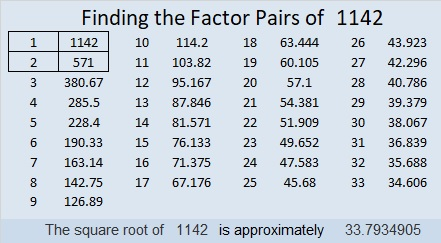# 1142 and Level 5

If you start in the right place, you will be able to solve this puzzle using logic and basic multiplication facts. Don’t get discouraged, if you make a mistake. I know you can figure this one out!Print the puzzles or type the solution in this excel file: 12 factors 1134-1147

Here are some facts about the number 1142:

• 1142 is a composite number.
• Prime factorization: 1142 = 2 × 571
• The exponents in the prime factorization are 1 and 1. Adding one to each and multiplying we get (1 + 1)(1 + 1) = 2 × 2 = 4. Therefore 1142 has exactly 4 factors.
• Factors of 1142: 1, 2, 571, 1142
• Factor pairs: 1142 = 1 × 1142 or 2 × 571
• 1142 has no square factors that allow its square root to be simplified. √1142 ≈ 33.793491142 is a palindrome in three different bases:
It’s 949 in BASE 11 because 9(11²) + 4(11) + 9(1) = 1142,
3G3 in BASE 17 (G is 16 base 10) because 3(17²) + 16(17) + 3(1) = 1142,
and 2H2 in BASE 20 (H is 17 base 10) because 2(20²) + 17(20) + 2(1) = 1142

This site uses Akismet to reduce spam. Learn how your comment data is processed.